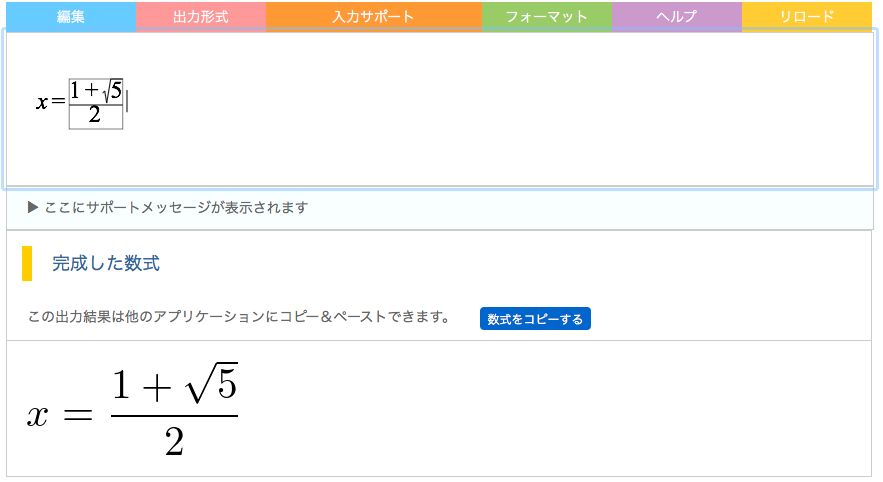### What is MathTOUCH?In 2011, we developed a math input editor, named MathTOUCH, that facilitates conversion from a colloquial-style mathematical text. In this method, the list of candidates for the desired mathematical expression is shown in WYSIWYG based on the userʼs input. After all the elements that a user wishes to use are chosen interactively, the desired expression can be formed. This method enables the user to input almost any mathematical formula without learning a new language or syntax.

### Try MathTOUCH!

MathTOUCH is a free online mathematical input editor service. Try it Now!